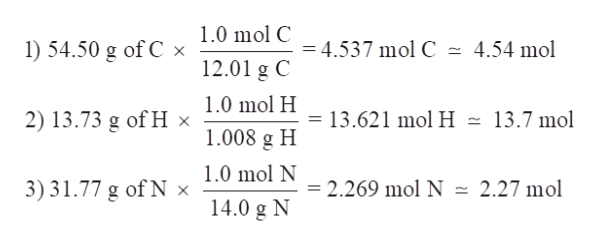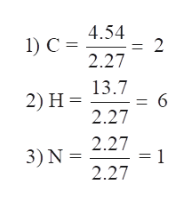# Part CThe rotten smell of a decaying animal carcass is partially due to a nitrogen-containing compound called putrescine. Elemental analysis of putrescine indicates that it consists of: 54.50% C, 13.73% H, and 31.77% N. Calculate the empirical formula of putrescine.Express your answer as a chemical formula.

Question
2 views

#### Part C

The rotten smell of a decaying animal carcass is partially due to a nitrogen-containing compound called putrescine. Elemental analysis of putrescine indicates that it consists of: 54.50% C, 13.73% H, and 31.77% N. Calculate the empirical formula of putrescine.
check_circle

Step 1

Consider 100 g of the compound is present,

C = 54.50 g

H = 13.73 g

N = 31.77 g

Now will convert the masses of the elements to moles by using the molar mass of each element –help_outlineImage Transcriptionclose1.0 mol C 1) 54.50 g of C x = 4.537 mol C - 4.54 mol 12.01 g C 1.0 mol H 2) 13.73 g of H × = 13.621 mol H - 13.7 mol 1.008 g H 1.0 mol N 3) 31.77 g of N x = 2.269 mol N = 2.27 mol 14.0 g N fullscreen
Step 2

Now divide the values of C, H and N by the smal...help_outlineImage Transcriptionclose4.54 1) C = 2.27 13.7 2) Н%— 2.27 2.27 = 1 3) N = 2.27 fullscreen

### Want to see the full answer?

See Solution

#### Want to see this answer and more?

Solutions are written by subject experts who are available 24/7. Questions are typically answered within 1 hour.*

See Solution
*Response times may vary by subject and question.
Tagged in

### Chemistry